# assuming equal concentrations, rank these solutions by pH acmillan learning Assuming equal concentrations, rank these solutions...

assuming equal concentrations, rank these solutions by pH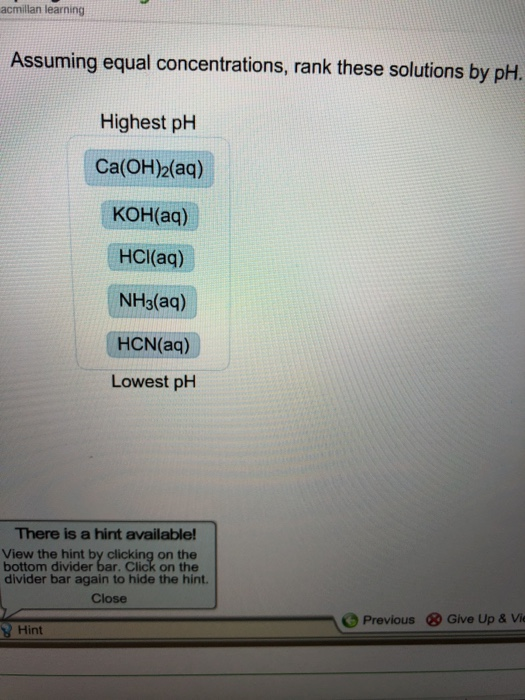acmillan learning Assuming equal concentrations, rank these solutions by pH. Highest phH ca(OH)2(aa) KOH(aq) HCI(aq) NH3(aq) HCN(aq) Lowest pH There is a hint available! View the hint by clicking on the bottom divider bar. Click on the divider bar again to hide the hint. Close Previous & Give Up & Vi Hint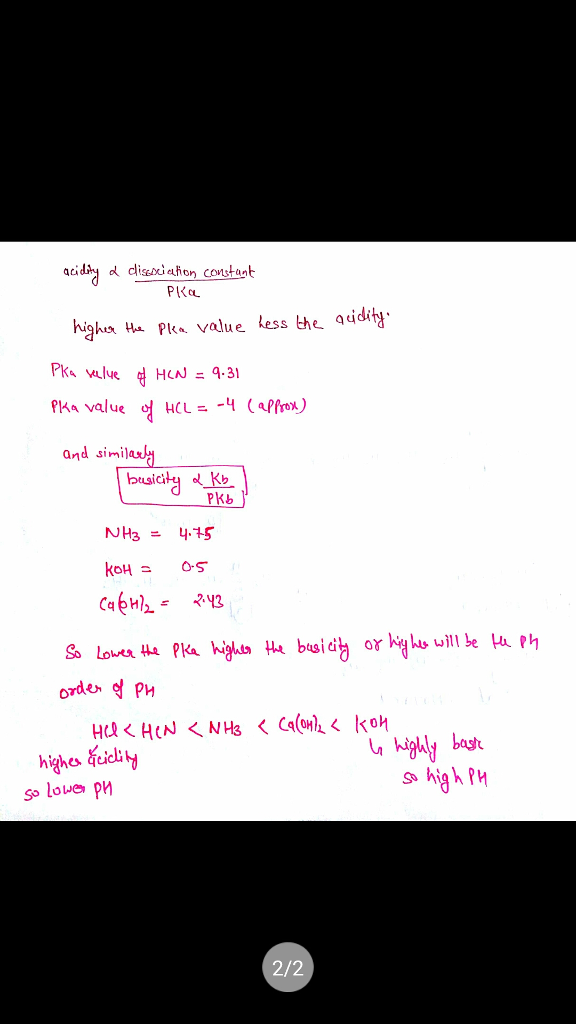#### Earn Coin

Coins can be redeemed for fabulous gifts.

Similar Homework Help Questions
• ### Assuming equal concentrations rank these solutions by pH Ca OH 2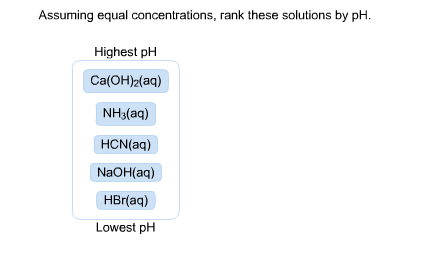Assuming equal concentrations, rank these solutions by pH. Highest pH Ca(OH)_2(aq) NH3(aq) HCN(aq) NaOH(aq) HBr(aq) Lowest pH

• ### assuming equal concentrations rank these solutions by PH Sr(OH)2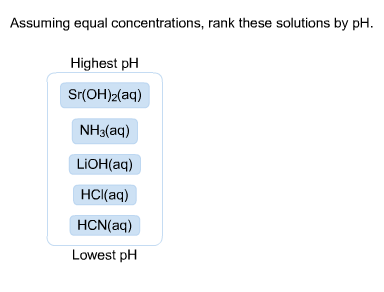Assuming equal concentrations, rank these solutions by pH. Assuming equal concentrations, rank these solutions by pH Highest pH Sr(OH)2(aq) NH3(aq) LiOH(aq) HCl(aq) HCN(aq) Lowest pH

• ### assuming equal concentrations rank these solutions by pH. HClO4

Assuming equal concentrations, rank these solutions by pH. Highest pH to Lowest pH. HClO4(aq) Sr(OH)2(aq) NH3(aq) KOH(aq) HF(aq)

• ### Assuming equal concentrations rank these solutions by pH HBr

Assuming equal concentrations, rank these solutions by pH from highest pH to lowest pH. Ca(OH)2, HF, NH3, NaOH, HBr. all aq

• ### assuming equal concentrations rank these solutions by pH. RbOH

Assuming equal concentrations, rank these solutions by pH. Highest pH(1) Lowest pH(5) RbOH(aq), Sr(OH)2(aq), HCl(aq), HF(aq), NH3(aq), from highest to lowest.

• ### Assuming equal concentrations rank these solutions by pH NaOH

Assuming equal concentrations, rank these solutions by pH. highest pH to lowest pH.. NaOH, HF, Ca(OH)2, NH3, HCL

• ### ning Sapling Learning Assuming equal concentrations, rank these solutions by pH. Highest pH Ca(OH)2(aq) HCl(aq) Incorrect....ning Sapling Learning Assuming equal concentrations, rank these solutions by pH. Highest pH Ca(OH)2(aq) HCl(aq) Incorrect. Acids have low pH. Identify the in this list and place it at the bott ranking NH3(aq) HF(aq) RbOH(aq) Lowest pH Week 8.1.pdf Failed Virus scan failed e to search DLL

• ### Assuming equal concentrations, rank these solutions by pH. Highest pH Lowest pH Ca(OH)2(aq) LiOH(aq) NH3(aq) HBrO(aq)...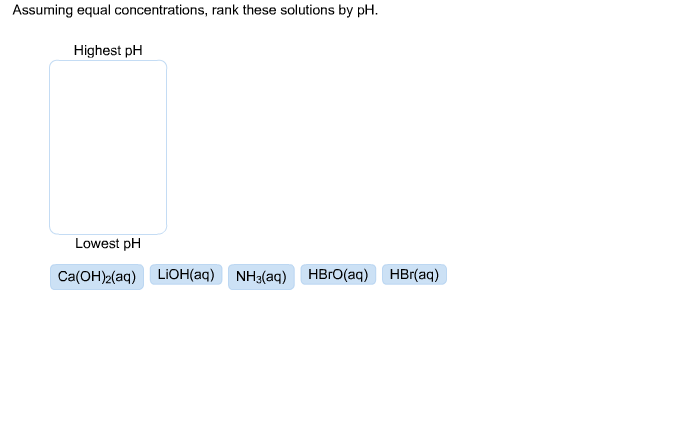Assuming equal concentrations, rank these solutions by pH. Highest pH Lowest pH Ca(OH)2(aq) LiOH(aq) NH3(aq) HBrO(aq) HBr(aq)

• ### 23. Assuming equal concentrations, rank these solutions by pH. Highest ph Lowest pH KOH(aq) AICl3(aq) KNO3(aq)...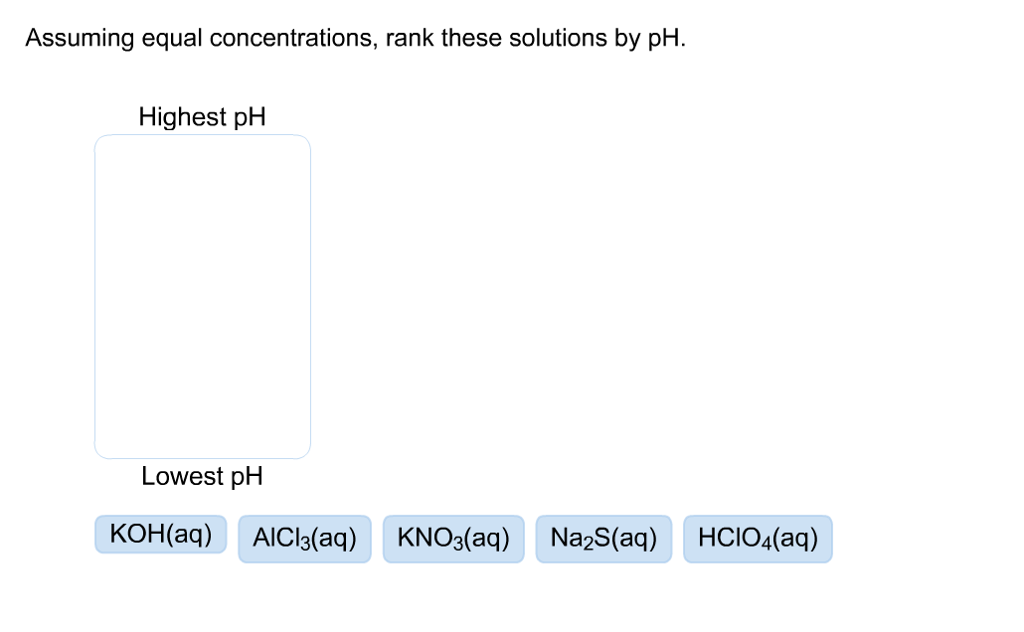23. Assuming equal concentrations, rank these solutions by pH. Highest ph Lowest pH KOH(aq) AICl3(aq) KNO3(aq) Na2S(aq) HCIO4(aq)

• ### Assuming equal concentrations, arrange these solutions by pH. Highest pHLowest pH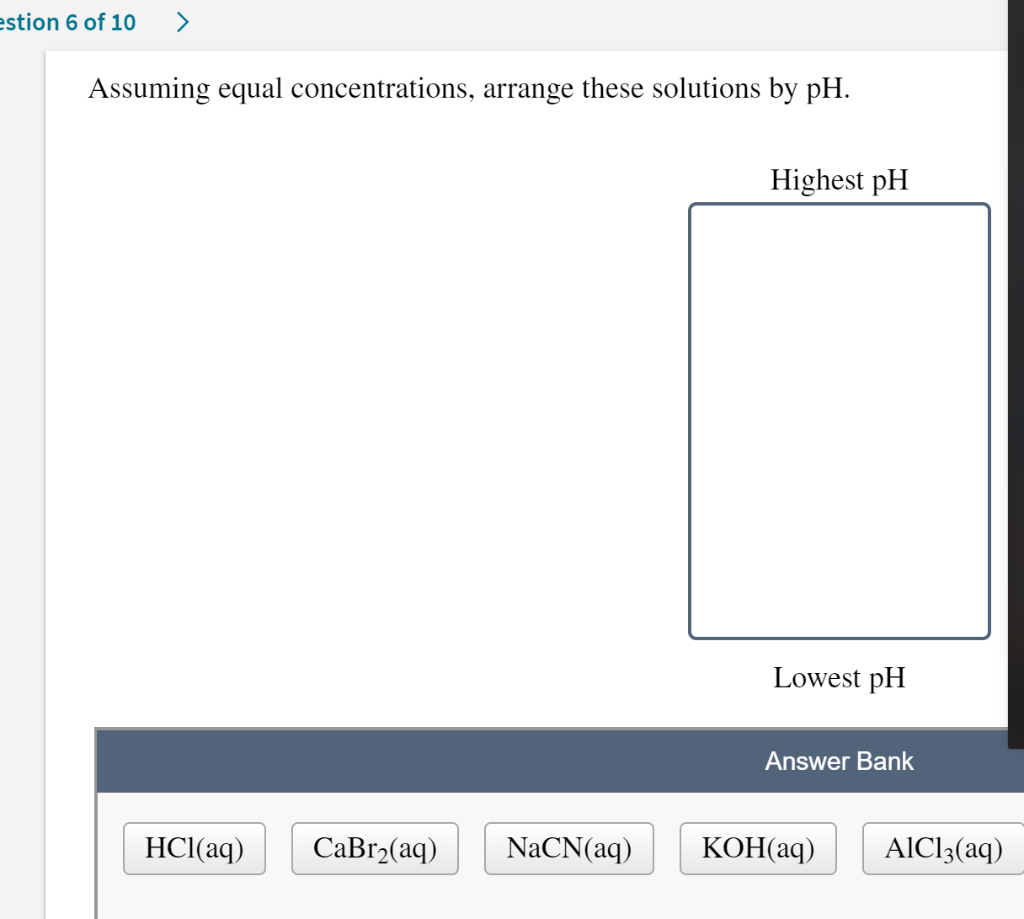Assuming equal concentrations, arrange these solutions by pH. Highest pHLowest pH stion 6 of 10> Assuming equal concentrations, arrange these solutions by pH Highest pH Lowest pH Answer Bank HCl(aq)CaBr2(aq)NaCN(aq)KOH(aq) AlCl3(aq) stion 6 of 10> Assuming equal concentrations, arrange these solutions by pH Highest pH Lowest pH Answer Bank HCl(aq)CaBr2(aq)NaCN(aq)KOH(aq) AlCl3(aq)